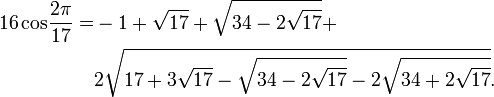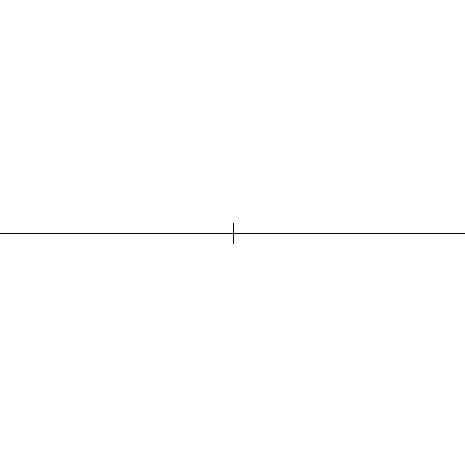## Sunday, 20 March 2011

### Gauss and Constuctable Polygons

By special request, for Steven Colyer who wrote, "I WILL point out one thing I wish you'd mentioned, and that was Gauss' accomplishment with the number 17. "

I assume he is talking about  Gauss' discovery that the heptadecagon was constructable with the classic tools of Greek Geometry.... hope that's it anyway.

So here is my version of that story, with many clips from Wolfram and Wikipedia...

One of the great problems of antiquity, along with doubling the cube and squaring the circle, was construction of a  heptagon, a seven-sided polygon, with a straight edge and compass.  Euclid had demonstrated the construction of the pentagon, the hexagon was easy, and so the early geometricians focused on the "next one". The early geometers knew that if an n-gon could be done, a 2n-gon was easy, so the octagon was easy, the decagon was easy, butt the nonagon also caused problems.  Over the years it became an open question in mathematics, "Which polygons are constructable with straight edge and compass?"

On April 30, 1796, Gauss, at the age of nineteen, proved the constructability of the regular 17-gon (heptadecagon).  He did this by showing, (I think) that it could be factored into equations involving no more than quadratics.    I have been looking on line for a copy of Disquisitiones Arithmeticae  in my price range (literally-free) but have not found one to see how he explains.  Within five years he had developed a theory that described exactly which polygons were, and which were not, constructable. He didn't actually construct one, but he proved it could be constructed. The proof relies on the property of irreducible polynomial equations that roots composed of a finite number of square root extractions only exist when the order of the equation is the product of powers of two and Fermat Primes.  Gauss showed that the 17-gon is constructable since the sine and cosine of  17 can be expressed with basic arithmetic and square roots alone (which can be formed with a compass and straightedge).   The first actual method of construction was devised by Johannes Erchinger, a few years after Disquisitiones Arithmeticae was published. In the book Gauss supposedly writes cos(2pi/17) as an expansion of square roots which would look like this in modern notation\begin{align} 16\,\operatorname{cos}{2\pi\over17} = & -1+\sqrt{17}+\sqrt{34-2\sqrt{17}}+ \\ & 2\sqrt{17+3\sqrt{17}- \sqrt{34-2\sqrt{17}}- 2\sqrt{34+2\sqrt{17}}}. \end{align}
(thank you wikipedia)(wikipedia)

Guass was so  proud of this, among all his great discoveries, that he told his close friend, Farkas  Bolyai, that the regular 17-gon should adorn his tombstone, but this was not done. There is a 17 pointed star on the base of a monument to him in Brunswick because the stonemason felt everyone would mistake the 17-gon for a circle.

Five years later, he developed the theory of Gaussian periods in his Disquisitiones Arithmeticae. This theory allowed him to formulate a sufficient condition for the constructability of regular polygons:
A regular n-gon can be constructed with compass and straightedge if n is the product of a power of 2 and any number of distinct Fermat primes.
Gauss stated without proof that this condition was also necessary, but never published his proof. A full proof of necessity was given by Pierre Wantzel in 1837.

### Detailed results by Gauss' theory

Only five Fermat primes are known:
F0 = 3, F1 = 5, F2 = 17, F3 = 257, and F4 = 65537 (sequence A019434 in OEIS)
The next twenty-eight Fermat numbers, F5 through F32, are known to be composite.
Thus an n-gon is constructable if
n = 3, 4, 5, 6, 8, 10, 12, 15, 16, 17, 20, 24, … (sequence A003401 in OEIS),
while an n-gon is not constructable with compass and straightedge if
n = 7, 9, 11, 13, 14, 18, 19, 21, 22, 23, 25, … (sequence A004169 in OEIS).

### Connection to Pascal's triangle

There are 31 known numbers that are multiples of distinct Fermat primes, which correspond to the 31 odd-sided regular polygons that are known to be constructable. These are 3, 5, 15, 17, 51, 85, 255, 257, … , 4294967295 (sequence A001317 in OEIS). As John Conway commented in The Book of Numbers, these numbers, when written in binary, are equal to the first 32 rows of the modulo-2 Pascal's triangle (see below), minus the top row. This pattern breaks down after there, as the 6th Fermat number is composite, so the following rows do not correspond to constructable polygons. It is unknown whether any more Fermat primes exist, and is therefore unknown how many odd-sided constructable polygons exist. In general, if there are x Fermat primes, then there are 2x−1 odd-sided constructable polygons.

Pascal's triangle mod-2
1  1   = 3
1  0  1  = 5
1  1  1  1  = 15
1  0  0  0   1 = 17
1  1  0  0    1   1  = 51
1  0  1  0   1   0  1 = 85
1  1  1  1  1    1   1  1  = 255   etc...

This biography of Gauss, by far the most comprehensive in English, is the work of a professor of German, G. Waldo Dunnington, who devoted most of his scholarly career to studying the life of Germany's greatest mathematician. The author was inspired to pursue this project at the age of twelve when he learned from his teacher in Missouri that no full biography of Gauss existed at the time. His teacher was Gauss's great granddaughter, Minna Waldeck Gauss. Long out of print and almost impossible to find on the used book market, this valuable piece of scholarship is being reissued in an augmented form with introductory remarks, an expanded and updated bibliography, and a commentary on Gauss's mathematical diary, by the eminent British mathematical historian, Jeremy Gray.

Steven Colyer said...

I assume he is talking about Gauss' discovery that the heptadecagon was constructable with the classic tools of Greek Geometry.... hope that's it anyway.

Yes that's it, and thank you very much Pat.

Currently I'm studying Fourier Analysis and Fourier Transforms, you know, the Calculus that explains how you can "schmeer" the discrete with the continuous, and vice versa, that is to say for one example: in equations (analysis), how one can express sines/cosines, sawtooth waves, and square waves in the same body of Mathematical tools. We studied this in Engineering school, but I forgot so it's a nice review.

I mention that because no matter how far one goes into analysis, geometry is the best way to hook interest in math (textbooks are the worst ... it's almost like if you want to kill a young student's love for a subject, force him to read a textbook on same), and Geometry will always be my greatest love.

Dave L. Renfro said...

When I get a chance to write it up (by tomorrow, maybe later today), I'll send you an algebraic way to obtain square root expressions for the 17th roots of 1.

For the time being, it may be useful to others to indicate what "constructible" means from an algebraic standpoint. Roughly speaking, the constructible numbers are those that can be obtained by solving at most quadratic equations (in series or in parallel, to use electrical circuit terminology), beginning with the integers. In this sense, the rational numbers are those that can be obtained by solving linear equations, beginning with the integers. [In the case of rational numbers, you can always manage with at most one linear equation, something that is not the case with the constructible numbers.]

Here are two examples, presented without proof.

One way to show that cos(pi/17) can be obtained by solving at most quadratic equations, beginning with the integers, is: Let b be the positive solution of 4x^2 + x - 1 = 0. Let c be the positive solution of x^2 + 4bx - 1 = 0. Let d be the negative solution of 4x^2 + (16b + 4)x - 4 = 0. Then cos(pi/17) is the negative solution of x^2 + cx + d = 0, divided by -2.

One way to show that cos(2*pi/17) can be obtained by solving at most quadratic equations, beginning with the integers (besides using appropriate trig identities with the previous sequence of steps), is: Let p, n be the positive and negative solutions of x^2 + x - 4 = 0. Let e be the positive solution of x^2 - px - 1 = 0. Let f be the positive solution of x^2 - nx - 1 = 0. Then cos(2*pi/17) is the greatest solution of x^2 - ex + f = 0.

Incidentally, I can think of two natural algebraic ways to define what it means for a complex number to be constructible. One way is exactly as above, which among other things implies that we can make use of any known constructible complex numbers as coefficients of quadratic equations in showing that some specified complex number is constructible. The other way is to use the above to define what it means for a real number to be constructible, and then define a + bi (where a, b are real numbers) to be constructible if and only if both a and b are constructible real numbers. It can be shown that these two definitions lead to the same collection of complex numbers.